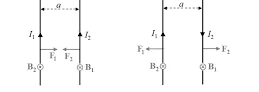#### Two long wires are hanging freely. They are joined first in parallel and then in series and then are connected with a battery. In both cases, which type of force acts between the two wires Option 1)  Attraction force when in parallel and repulsion force when in series   Option 2)  Repulsion force when in parallel and attraction force when in series   Option 3) Repulsion force in both cases   Option 4) Attraction force in both casesAs we learnt in

Force between two parallel current carrying conductors -- whereinI1 and I2 current carrying two parallel wires

a-seperation between two wires

When they are connected in parallel the current flowing through both are in same direction hence they attract each other. when they are in parallel they will tend to repel each other

Option 1)

Attraction force when in parallel and repulsion force when in series

correct

Option 2)

Repulsion force when in parallel and attraction force when in series

incorrect

Option 3)

Repulsion force in both cases

incorrect

Option 4)

Attraction force in both cases

incorrect